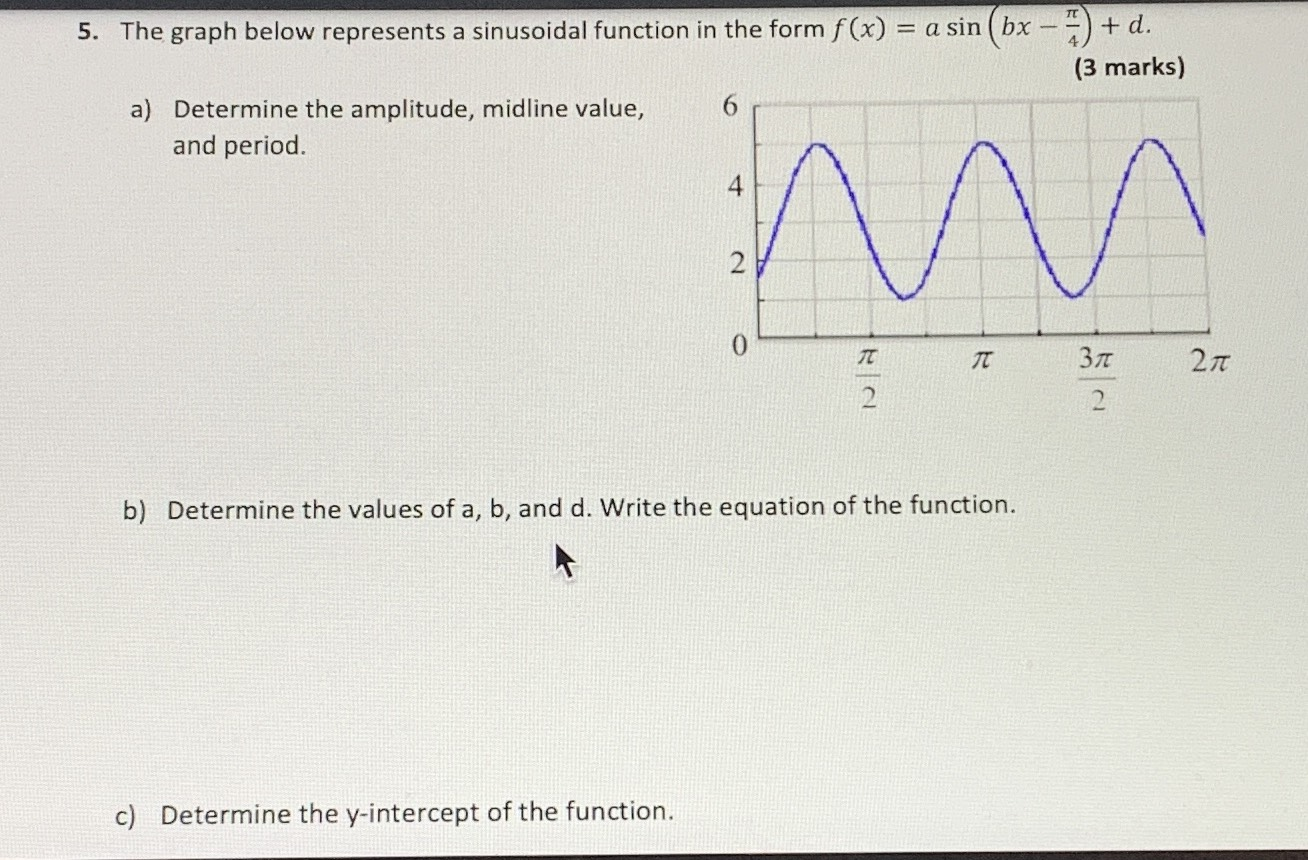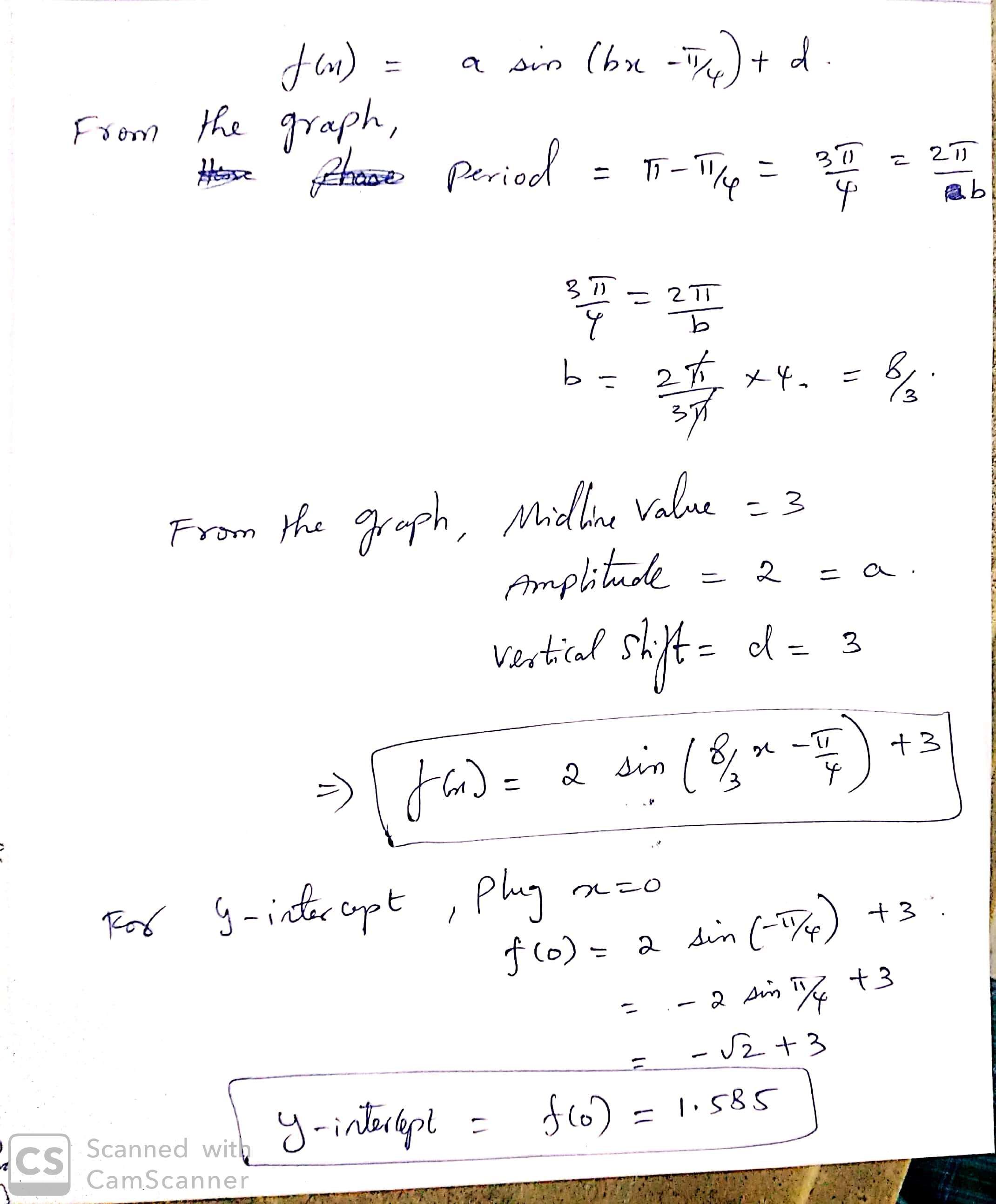### ¿Todavía tienes preguntas de matemáticas?

Pregunte a nuestros tutores expertos
Algebra
Pregunta5. The graph below represents a sinusoidal function in the form $$f ( x ) = a \sin ( b x - \frac { \pi } { 4 } ) + d$$ a) Determine the amplitude, midline value, and period. b) Determine the values of $$a , b$$ , and d. Write the equation of the function. c) Determine the $$y$$ -intercept of the function.